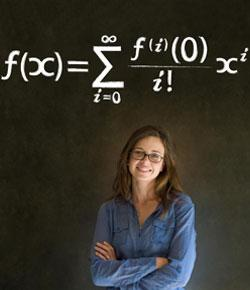# Maths Quiz For Grade 2

8 Questions | Total Attempts: 328SettingsThis quiz is for kids aged 6-8. The aim of the quiz is to test basic skills in numbers, addition, subtraction, multiplication and division.

• 1.
______ comes just before 413.
• A.

403

• B.

412

• C.

410

• D.

414

• 2.
In 289, the place value of 8 is____________.
• A.

Ones

• B.

Hundreds

• C.

Tens

• D.

Thousands

• 3.
Three hundred and one can be written as ______.
• A.

31

• B.

310

• C.

301

• D.

3001

• 4.
232, 242, ____ , 262. The missing number in the pattern is ____.
• A.

222

• B.

225

• C.

252

• D.

243

• 5.
There are 3 sweets in one packet. How many sweets will be there in 3packets?
• A.

12

• B.

27

• C.

9

• D.

3

• 6.
546 is one more ________.
• A.

545

• B.

446

• C.

646

• D.

746

• 7.
There are 4 apples in each basket. How many apples will be there in 2 baskets?
• A.

5

• B.

8

• C.

9

• D.

10

• 8.
Which one of the following is correct?
• A.

4+2 = 6

• B.

4+2 = 9

• C.

2+6 = 1

• D.

6+2 = 12Back to top